Mechanics Index

Introduction to Kinematics

Introduction

Kinematics deals with the motion of bodies and requires consideration of the geometry and time.  The forces involved in the motion of body are not considered in this area of work.

The notes on this page include use of vectors. The necessary background information is provided on page Vectors

Basics

 Time..The absolute measure of the orderly succession of events. Unit of time is the second Particle..a body is assumed with negligible dimensions Rigid body..A body with all internal points fixed relative to each other

Nomenclature

 s = distance travelled in time -(m) v = average velocity - (m/s) a = acceleration - (m/s2) t = time - (s) u = initial velocity - (m/s) v = final velocity - (m/s) θ = Rotation angle - (radians) ω 1 = Initial angular velocity -rads/s ω 2 = final angular velocity - rads /s n = angular speed revs/min α = Angular Acceleration rads/s 2

Particle Kinematics.

This discipline of mechanics deals with the displacement of particles over time without reference to the forces that cause the motion, velocity and acceleration of the particle.

Linear Motion..

Considering a particle moving along a path is space from position A to position B over a time interval.    The position vector r locates the particle relative to the reference frame (say cartesian xyz) .   The distance the particle moves along the path is designated s.    The change in position of the particle over the time is its linear displacement and is identified by the vector ( r B - r A )

The speed of the particle over a time period = (s 2 - s 1 ) /(t 2 - t 1 ) = the speed of the particle

As the time interval reduces to zero the change in position Δ r /Δ t = ( rB - rA ) / (t2 - t1 ) => dr/dt = v = the instantaneous velocity   The instantaneous velocity is tangential to the particle path

The derivative (with respect to time) of the instantaneous velocity is d 2r /dt 2 = the instantaneous acceleration.

Textbook formulae..Linear motion subject to uniform acceleration.

v = u + at

s = ut + a.t2 /2

s = (u + v) /2

v 2 = u 2 + 2.a.s

Using vector algebra....

 As an example of straight line motion consider a particle moving with a constant acceleration in the x direction.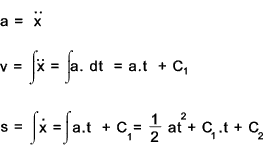C1 is a constant determined from the velocity at t = 0: Normally assuming initial velocity = u then C1 = u C2 is a constant determined from the displacement at t = 0: Normally assuming initial displacement = 0 then C2 = 0

Angular Motion..

A point rotates from A to B around a center C through a small angle θ in a time t .   As the time interval approaches zero the instantaneous angular velocity d θ /dt = ω . This angular velocity is a vector cross product and is shown in the direction as indicated below..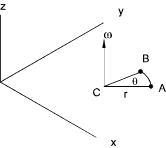The velocity of the point is obtained as a vector product of the angular velocity and the radius

v = ω x r

Angular velocity vectors are free vectors but they are not commutative..

Textbook formulae.. Angular motion subject to uniform acceleration

ω = 2.π .n /60

ω 2 = ω 1 + α . t

θ = ( ω 2 + ω 1 ) . t /2

ω 22 = ω 12 + 2 . α . s

θ= ω 12 + α . t 2 /2

Motion in a circle..

A particle moving in a circle of radius (r) at a constant velocity (v) and constant angular velocity of ω = v /r is accelerating towards the centre of the circle at a constant rate of v 2 /r = ω 2 . r.   The acceleration is primarily due to the rate of change of angular position of the particle...

An example of linear motion is that of a particle moving at a fixed velocity in a circular motion..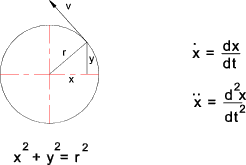Differentiate with respect to time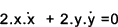Differentiate again with respect to time using ( d(uv) = u.dv + v du )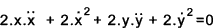Relating the Tangential velocity to the x an y velocity.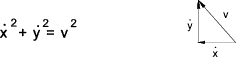Introducing velocity into equation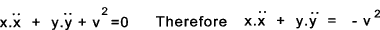Finally establishing formula for accelaration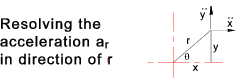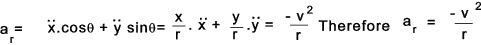Absolute and relative motion..

A reference frame can be fixed or relative.  In Newtonian mechanics the fixed reference frame is the primary inertial system in which there is zero absolute motion in space.   This is entirely impractical because it would have to take into account the movements of the earth relative to the sun and the sun in the universe etc. A reference frame based on the sun is called a Heliocentric reference frame and this again is impractical for normal mechanical engineering.   A practical reference frame is one based on the earth. This is called a geocentric reference frame and is sufficient as an inertial frame for normal engineering. Therefore when we identify the velocity of a vehicle or the acceleration of an object they are generally relative to the earths motion.

When an object is moving relative to a fixed reference frame it is called absolute motion.  When it is moving relative to a reference frame that is moving it is called relative motion.  An example of relative motion is someone moving in a travelling train or car.

Rotating unit vector

Consider a rotating unit vector e..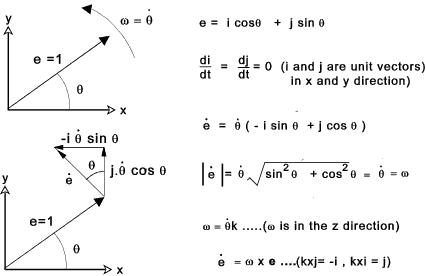Vector of Point in a rotating reference frame

A point P with a vector position r is moving in the xy plane. The position of this vector is identified in respect to unit vectors e 1 and e 2 fixed to a reference frame rotating at and angular velocity ω .. r 1 and r 2 are components of the motion in the e 1 and e 2 directions.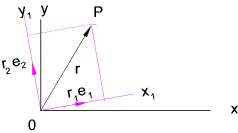The position is expressed as follows..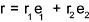The derivative of this equation with respect to time results in.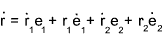It is shown above that de/dt = ω x e therefore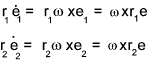Rewriting the equation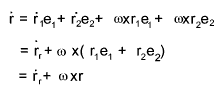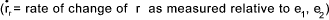Velocity of a point in a moving reference frame

A point P moving in the xy frame as shown below has a position vector of

r p = r o' + r

ro' locates O' relative to the fixed xy axes and r locates the point P relative to a axes (x 1,x 2)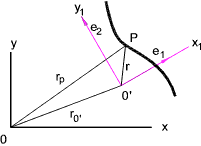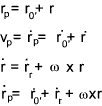If the moving frame is not rotating ω = 0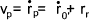Acceleration of a Particle

Differentiating (with respect to time) the equation for velocity (v) results the equation for accelaration (a)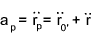Following the principles established above...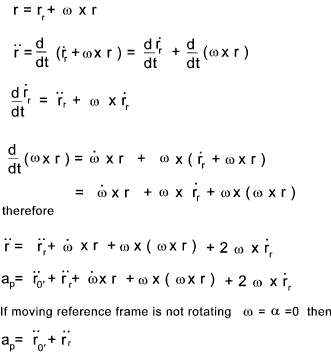Note : The term .....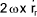..... is called the coriolis acceleration .
This represents the difference between the acceleration of P relative to O' as measured from the rotating and the non-rotating axes.

Rigid Body Kinematics in one plane.

Rigid body..A body with all internal points fixed relative to each other.  Three co-ordinates are required to determine the position and orientation of a body in plane motion.    A rigid body has three degrees of free in plane motion.
Definitions..

 Rectilinear Translation...All point on a rigid body move in a straight line Curvilinear Translation... The orientation of all points in a rigid body remain fixed while the body moves along a curved path Rotation about a fixed line.. All points in a rigid body move in a circular motion about a fixed line Plane motion...Each point in rigid body moves in a path parallel to a fixed plane

Plane motion for a rigid body can be easily developed from the equations for motion of a particle.   They are the same equations for the motion of a point but as all points are fixed relative to each other the term involving velocities and accelerations of points relative to each other are zero.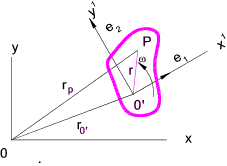The equations for velocity and acceleration when the reference axes x',y' are fixed to the rigid body ,are as follows...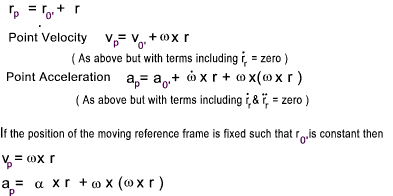Important Note:

It can be easily proved that whichever position on the rigid body is selected for the reference position O' the angular velocity and angular acceleration vectors are not changed.

Instantaneous Centre of Rotation

For any body moving in space the motion can be defined from the position, velocity, acceleration of any point on the body with the angular velocity and acceleration.  There is a point for this body for which the instantaneous translational velocity is zero i.e only rotation of the body about the point is occuring.  This point is called the instantaneous center of rotation and its location relative to the body is dependent on the relative values of the linear and angular velocities   For near zero angular velocities (near translation motion) the location is at near infinity.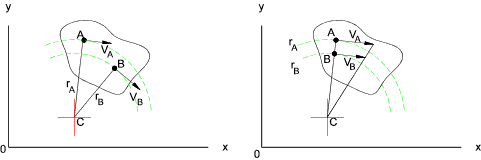The location of the instantaneous centre of rotation is easy to locate if the velocity and angular velocity of a point are known

If the location of a point and its velocity and angular velocity are known.
Considering point A the point C is on a line passing through A normal to the direction of the velocity.   rA = VA / ω

If the location of two points and their velocities
The point C is at the intersection of the lines drawn normal to the velocities of each of the points.
If the lines are co-incident then radius is established by geometry.. AC = AB.(VA) /(VA-VB)

Rigid Body kinematics in 3 Dimensions

Introduction

A particle requires three points to specify its position relative to the selected coordinate system and is therefore identified as having 3 degrees of freedom .  For a rigid body the location of three separate points of the body relative to the selected co-ordinate system are required.   Because the points are fixed relative to each other only six independent co-ordinates are required to locate the body is space and a unrestrained rigid body is therefore said to have six degrees of freedom.   e.g. A rigid body may be positioned by locating the position of one point of the body ( 3 co-ordinates), then positioning a line on the body (2 co-ordinates) and finally identifying a rotation about the line (1 co-odinates).   This sums up to 6 co-ordinates

The motion of a rigid body in three dimension can have a number of modes..

 Rectilinear Translation...All points on the rigid body move in a straight line Curvilinear Translation... The orientation of all points in a rigid body remain fixed while the body moves along a curved path Rotation about a fixed axis.. All points in a rigid body move in a circular motion about a fixed axis Rotation about a fixed point.. All points in a rigid body move in a circular motion about a fixed point General motion...The rigid body motion includes translation and rotation about Rotation Notes. If a rigid body is rotated from a position "A" through an angle of π/2 about the x axis and then rotated π/2 about the y axis it has been moved to a certain position "B".   If the same rigid body is rotated from a position "A" through and an angle of π/2 about the y axis and then is rotated π/2 about the x axis it has been moved to a position "C" which is not the same as "B".   Finite rotation does not obey the laws of vector addition
Euler & Chasles;'s Theorems

Euler's Theorem

If a point on a rigid body does not change its position then any series of successive rotations can be compounded to a rotation about a single axis

Chasles's Theorem

Any displacement of a rigid body may be compounded from a single rotation about any selected point plus a translation of that point.

A third theorem ( Poinsot's Central axis theorem) is also provided..

Any finite displacement of a rigid body may be reduced to a single rotation about an axis plus a translation parallel to the same axis.  This theorem only relates the displacement and not to the paths taken by points.

These theoerems can be applied to angular velocities

Any motion of a rigid body can be described by a single angular velocity plus a translational velocity parallel to the angular velocity vector.

Any motion of a body about a point may be represented by a single angular velocity about an axis through that point.

Any motion of a rigid body my be represented by the velocity of a point plus the angular velocity about an axis passing through the points...

Rectilinear Translation.& Curvilinear Translation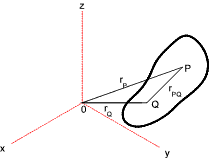All points on the body will move in parallel straight or curve lines.  There is no relative velocity or acceleration between any points on the body.

Position... r P = r Q + r PQ

Velocity...v P = v Q + v PQ
Acceleration...a P = a Q + a PQ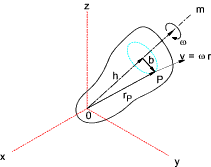Rotation of a rigid body about fixed axis is shown in the diagram.   The vector of the rotary motion has sense and direction in accordance with the right hand rule which is fixed along the direction of the axis.  There is zero velocity due to rotation at the axis.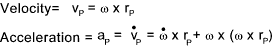Rotation of a rigid body about a fixed point can always be reduced to rotation of a body about an instantaneous axis of rotation.

Considering for example a rigid body (cone) which is rotating about a horizontal axis (shaft),angular velocity = ω1,and the shaft is itself rotating about a vertical axis, angular velocity = ω2.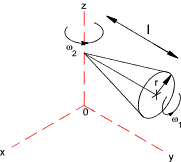If ω2 = 0 then the axis rotation is the centre line of the shaft and the velocity of any point on the shaft is proportional to the radius (max = r)from the shaft.  If the rotation about the vertical axis is increased to a certain value the the velocity of the shaft axis is proportional to ω2 x the shaft length (max = l) from the axis (radius).   If the two angular velocities are the same and the radius of the cone r = l then at any instant the velocity of top surface of the cone is zero. i.e the top surface is the instantaneous axis of rotation.

This grossly simplified representation illustrates the principle .. if ω2 is increased the body cone representing the path of the instantaneous axis of rotation will obviously be larger than the actual surface of the cone.

For the model illustrated there is also a space cone which is the path the instantaneous axis or rotation follows in space- (an inverted cone centered the vertical axis )..

For the example above the instantaneous angular velocity of the cone =

ω = ω1 + ω2 = ω1i + ω2j

In the real world the motions and shapes are not as shown but the interaction between the body rotation on its axis and its angular motion is space still results in an instantaneous axis of rotation between a instantaneous body cone and an instantaneous space cone.   The body cone may rotate outside or inside the space cone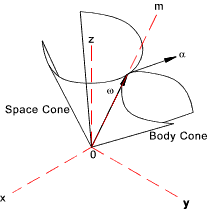One determining the instantaneous angular velocity the angular acceleration α is tangential to the contact point of the two cones as shown. The velocity and acceleration at any point is simply determined as shown below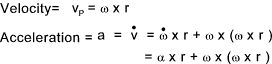General Motion.

The general case of 3D motion of rigid bodies reduces to translation + rotation about a fixed axis.  This is basically a generalisation of the theorems provided above.

For a body possessing linear plus angular motion it is often not possible to have an instantaneous axis of rotation because all points may have non-zero velocities.   The most convenient method of kinematic analysis of rigid bodies in space 3 dimensions is by using the principles of relative motion.   The primary (absolute)reference system XYZ are supplemented with a reference system(xyz) attached to some point on the rigid body.
The attached reference system may be a translating one or a rotating one.

Translating reference system
This motion simply develops the motions already studied in the previous section for particle motions and rigid body motions. The basic motion equations are :

r P = r Q + r PQ
v P = v Q + v PQ
a P = a Q + a PQ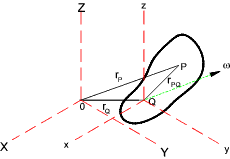The above equations are sufficient if the angular velocity ω is zero however if this is not the case the following equations for velocity and acceleration are more definitive;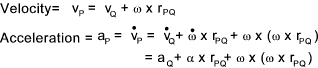Rotating reference system

A more general form of the relative reference axis method uses rotating reference axes.  The reference axes xyz are rotating with an angular velocity of Ω. The rigid body has a rotation velocity ω as before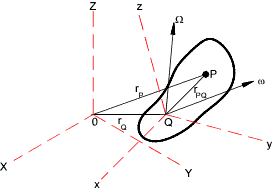The basic motion equations are as above.  The expression for velocity and acceleration of point P are provided below.  The derivation is a simple extension of that provided above for 2 Dimensional motion with a rotating relative axis with the third dimension (z) added.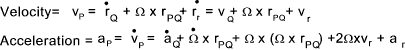v r and a r = are the velocity and acceleration of P relative to the rotating xyz axis.

Note:

The equations above are based on the general case of the angular velocity of the rigid body (ω)is different to the angular velocity of the axis ( Ω). If the reference axis is fixed to the body then ω will equal Ω and vr and a r will equal zero... For this case the formula will be the same as for the translating reference axis above..

 Links to Kinematics Physics - Kinematics..An unusual but useful information source Rigid body dynamics..Chris Hecker.. Set of articles for designers of realistic games - Good dynamics analysis info. Basic kinematics..A download Basic but clear information.. Kinematics -Wikipedia..Detailed informative notes..

Mechanics Index